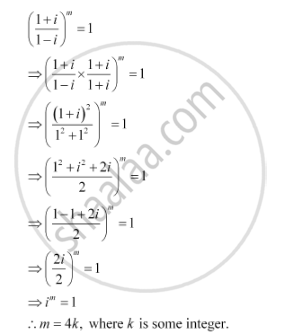CBSE (Arts) Class 11CBSE
Share

# If ((1+I)/(1-i))^M = 1, Then Find the Least Positive Integral Value Of M. - CBSE (Arts) Class 11 - Mathematics

ConceptAlgebra of Complex Numbers

#### Question

if ((1+i)/(1-i))^m =   1, then find the least positive integral value of m.

#### SolutionTherefore, the least positive integer is 1.

Thus, the least positive integral value of m is 4 (= 4 × 1).

Is there an error in this question or solution?

#### Video TutorialsVIEW ALL 

Solution If ((1+I)/(1-i))^M = 1, Then Find the Least Positive Integral Value Of M. Concept: Algebra of Complex Numbers.
S